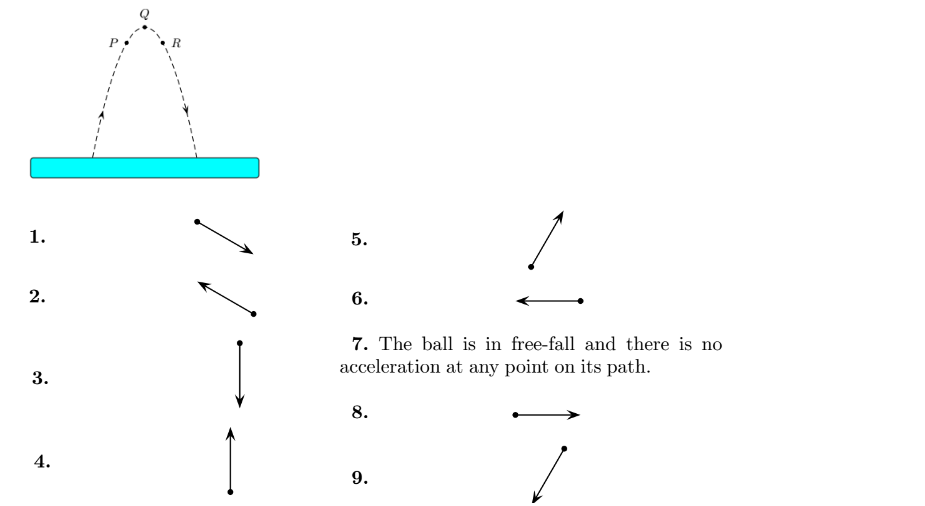# Problem: A ball is thrown and follows the parabolic path shown. Air friction is negligible. Point Q is the highest point on the path. Points P and R are the same height above the ground. Which of the following diagrams best indicates the direction of the acceleration, if any, on the ball at point P?

97% (81 ratings)
###### Problem Details

A ball is thrown and follows the parabolic path shown. Air friction is negligible. Point Q is the highest point on the path. Points P and R are the same height above the ground. Which of the following diagrams best indicates the direction of the acceleration, if any, on the ball at point P?